Open in App
Not now

# Class 9 RD Sharma Solutions – Chapter 15 Areas of Parallelograms and Triangles- Exercise 15.3 | Set 1

• Last Updated : 19 Apr, 2021

### Question 1. In the figure, compute the area of quadrilateral ABCD.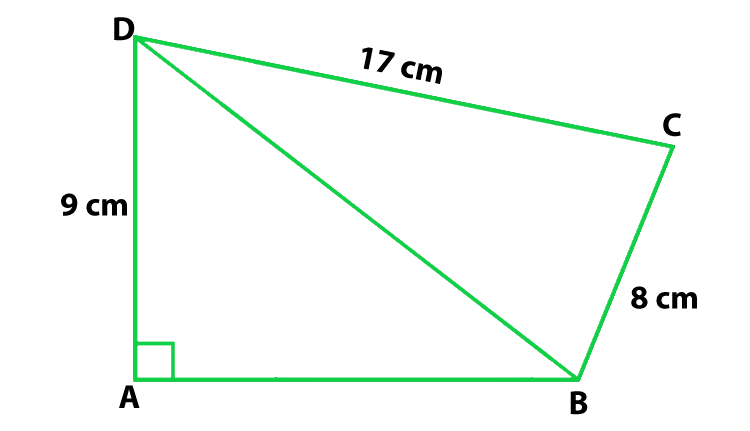Solution:

According to the question

DC = 17 cm, AD = 9 cm and BC = 8 cm

Find: the area of quadrilateral ABCD

In ΔBCD,

Using Pythagoras Theorem

CD2 = BD2 + BC2

172 = BD2 + 82

BD2 = 289 − 64

BD = 15

So, the area of ΔBCD = 1/2(8 × 17) = 68

In ΔABD

Using Pythagoras Theorem

152 = AB2 + 92

AB2 = 225 − 81 = 144

AB = 12

So, the area of ΔABD = 1/2(12 × 9) = 54

Now we find the ar(quad ABCD) = ar(ΔABD) + ar(ΔBCD)

ar(quad ABCD) = 54 + 68 = 122 cm2

Hence, the area of quadrilateral ABCD is 122 cm2

### Question 2. In the figure, PQRS is a square and T and U are, respectively, the midpoints of PS and QR. Find the area of ΔOTS if PQ = 8 cm.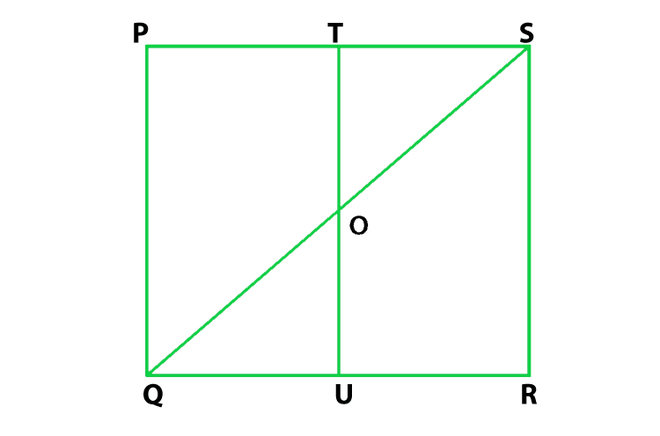Solution:

According to the question

T and U are mid-points of PS and QR

Hence, TU ∥ PQ

PQ = 8 cm

Find: the area of ΔOTS

In ΔPQS,

It is given that T is the midpoint of PS and TO ∥ PQ

therefore, TO = (1/2) PQ = 4 cm

and TS = (1/2) PS = 4 cm

So, the area of ar(ΔOTS) = (1/2)(TO × TS)

= (1/2)(4 × 4) = 8 cm2

Hence, the area of ΔOTS is 8 cm2

### Question 3. Compute the area of trapezium PQRS in figure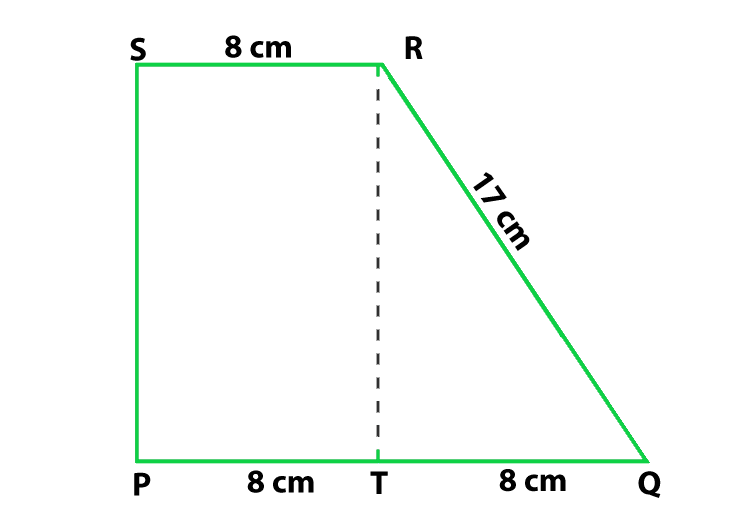Solution:

According to the figure

PQ = 16 cm

Here T is the mid-point of side PQ so

PT = QT = 8 cm

SR = 8 cm

RQ = 17 cm

Find: the area of trapezium PQRS

So, the ar(trap. PQRS) = ar(rect. PSRT) + ar(ΔQRT)  ….(1)

So, In ΔQRT

Using Pythagoras Theorem

QR2 = QT2 + RT2

RT2 = QR2 − QT2

RT2 = 172 − 82 = 225

RT = 15

So, the area of ΔQRT

= 1/2(QT × RT) = 8 × 15 = 180/2 = 60

Now we find the area of rectangle PSRT

= PT × RT = 8 × 15 = 120

Now put all these values in eq(1), we get

ar(trap. PQRS) = 120 + 60 = 180

Hence, the area of trapezium is 180 cm2

### Question 4. In figure, ∠AOB = 90°, AC = BC, OA = 12 cm and OC = 6.5 cm. Find the area of ΔAOB.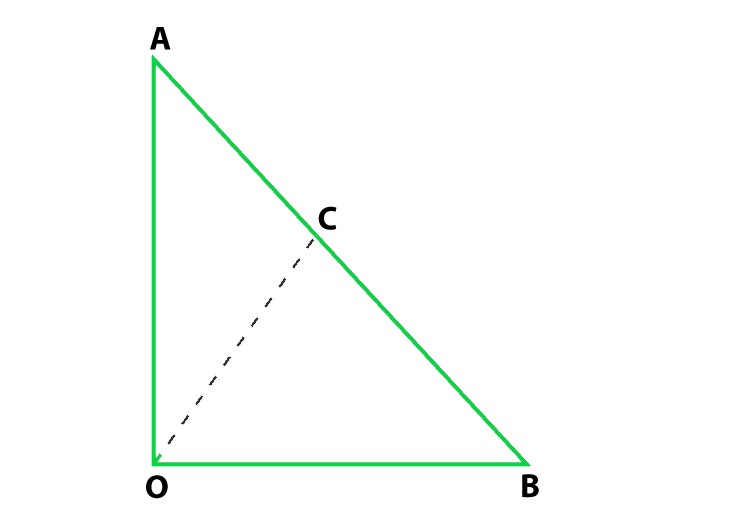Solution:

According to the question

∠AOB = 90°, AC = BC, OA = 12 cm and OC = 6.5 cm

Find: the area of ΔAOB

From the figure, C is the mid-point of hypotenuse AB so in right triangle

the mid-point is equidistant from the vertices

therefore, CA = CB = OC

CA = CB = 6.5 cm

AB = 13 cm

In ΔOAB,

Using Pythagoras Theorem

AB2 = OB2 + OA2

132 = OB2 + 122

OB2 = 132 − 122 = 169 − 144 = 25

OB = 5

Now we find the area of ΔAOB = (1/2)(12 × 5) = 30 cm2

Hence, the area of ΔAOB is 30 cm2

### Question 5. In figure, ABCD is a trapezium in which AB = 7 cm, AD = BC = 5 cm, DC = x cm, and distance between AB and DC is 4 cm. Find the value of x and area of trapezium ABCD.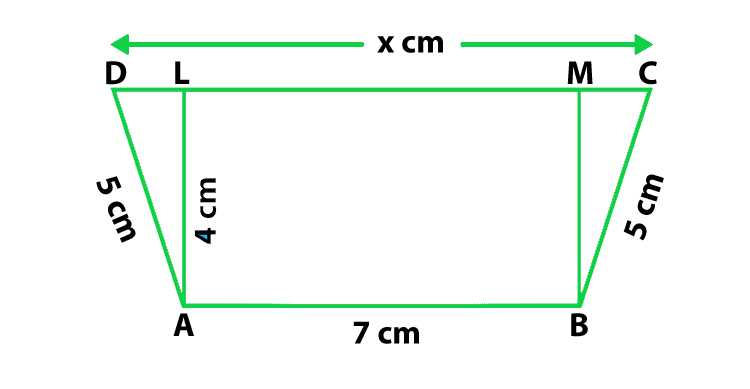Solution:

According to the question

AB = 7 cm, AD = BC = 5 cm

Construction: Draw AL ⊥ DC, BM ⊥ DC,

AL = BM = 4 cm and LM = 7 cm

Find: the value of x and area of trapezium ABCD.

Using Pythagoras Theorem

25 = 16 + DL2

DL = 3 cm

Similarly,

MC = √BC2 – BM2 = √25 – 16 = 3 cm

So, x = CD = CM + ML + LD = (3 + 7 + 3) cm = 13 cm

Now we find the area of trapezium ABCD = 1/2(AB + CD) × AL

= 1/2(7 + 13) × 4 cm2 = 40 cm2

Hence, the value of x is 3 cm and the area of trapezium is 40 cm2

### Question 6. In figure, OCDE is a rectangle inscribed in a quadrant of a circle of radius 10 cm. If OE = 2√5 cm, find the area of the rectangle.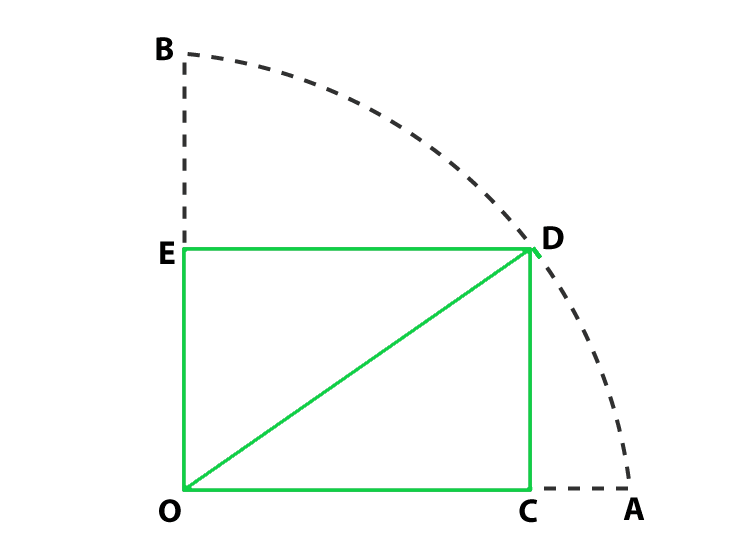Solution:

According to the question

OD = 10 cm and OE = 2√5cm

Find: the area of the rectangle OCDE

So, In ΔDEO

By using Pythagoras theorem

OD2 = OE2 + DE2

DE = √OD2 – OE2

= √102 – (2√5)2 = 4√5 cm

Now we find the area of rectangle OCDE = OE × DE

= 2√5 x 4√5 cm2 = 40 cm2

Hence, the area of rectangle is 40 cm2

### Question 7. In figure, ABCD is a trapezium in which AB ∥ DC. Prove that ar(ΔAOD) = ar(ΔBOC)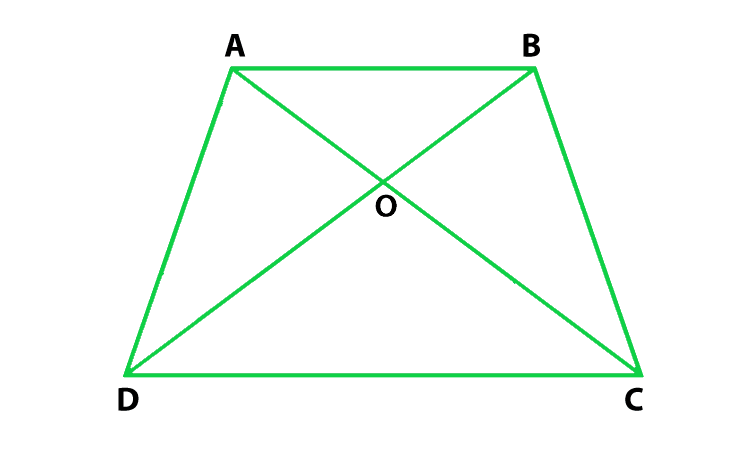Solution:

According to the question

ABCD is a trapezium in which AB ∥ DC

To prove : ar(ΔAOD) = ar(ΔBOC)

Proof :

According to the figure, we conclude that ΔADC and ΔBDC are on the

same base DC and between same parallels AB and DC

Here, ar(ΔADC) = ar(ΔAOD) + ar(ΔDOC)

Similarly, ar(ΔBDC) = ar(ΔBOC) + ar(ΔDOC)

Now put all these values in eq(1), we get

ar(ΔAOD) + ar(ΔDOC) = ar(ΔBOC) + ar(ΔDOC)

ar(ΔAOD) = ar(ΔBOC)

Hence, proved

### Question 8. In figure, ABCD, ABFE and CDEF are parallelograms. Prove that ar(ΔADE) = ar(ΔBCF).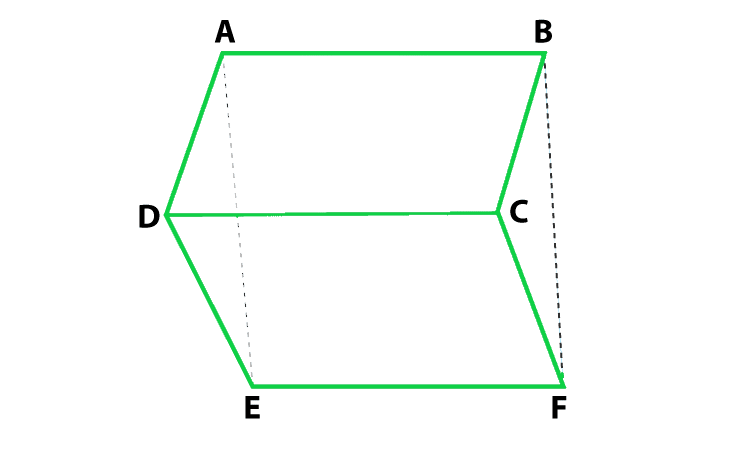Solution:

According to the question

ABCD is parallelogram, so, AD = BC

CDEF is parallelogram, so, DE = CF

ABFE is parallelogram, so, AE = BF

Proof:

DE = CF

AE = BF

So, by SSS congruence,

Hence, proved

### Question 9. Diagonals AC and BD of a quadrilateral ABCD intersect each other at P. Show that: ar(ΔAPB) × ar(ΔCPD) = ar(ΔAPD) × ar(ΔBPC).

Solution:

Construction : Draw BQ ⊥ AC and DR ⊥ AC

Prove: ar(ΔAPB) × ar(ΔCPD) = ar(ΔAPD) × ar(ΔBPC)

Proof:

Lets take L.H.S

= ar(ΔAPB) × ar(ΔCDP)

= (1/2) [(AP × BQ)] × (1/2 × PC × DR)

= (1/2 × PC × BQ) × (1/2 × AP × DR)

= ar(ΔAPD) × ar(ΔBPC)

L.H.S = R.H.S

Hence proved

### Question 10. In figure, ABC and ABD are two triangles on the base AB. If line segment CD is bisected by AB at O, show that ar(ΔABC) = ar(ΔABD).Solution:

According to the question

ABC and ABD are two triangles on the base AB

and CD is bisected by AB at O,

To prove: ar(ΔABC) = ar(ΔABD).

Construction: CP ⊥ AB and DQ ⊥ AB.

Proof:

First we find the area of ΔABC = 1/2 × AB × CP         …..(i)

Now we find the area of ΔABD = 1/2 × AB × DQ              …..(i)

So, in ΔCPO and ΔDQO,

∠CPO = ∠DQO           (Each 90°)

CO = OD                    (Given)

∠COP = ∠DOQ                           (Vertically opposite angles are equal)

So, by AAS congruency

ΔCPO ≅ ΔDQO

So, by C.P.C.T

CP = DQ        …..(iii)

So, from equation (i), (ii), and (iii)

ar(ΔABC) = ar(ΔABD)

Hence proved

My Personal Notes arrow_drop_up
Related Articles